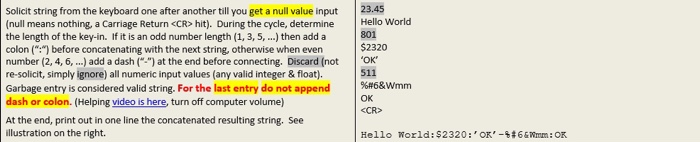# Programming language in python Solicit string from the keyboard one after another till you get a...

###### Question:

programming language in pythonSolicit string from the keyboard one after another till you get a null value input (null means nothing a Carriage Return <CR> hit). During the cycle, determine the length of the key-in. If it is an odd number length (1, 3, 5, ...) then add a colon (":") before concatenating with the next string, otherwise when even number (2, 4, 6, ...) add a dash (") at the end before connecting. Discard (not re-solicit, simply ignore) all numeric input values (any valid integer & float). Garbage entry is considered valid string. For the last entry do not append dash or colon. (Helping video is here, turn off computer volume) At the end, print out in one line the concatenated resulting string. See illustration on the right. 23.45 Hello World 801 $2320 'OK' 511 %6& Wmm <CR> Hello World:$2320:'OK'-4366 mm: OK

#### Similar Solved Questions

##### Question 3 (1 point) Given the following informtion: Long term assets = $350,000, Revenues =$1,200,000,...
Question 3 (1 point) Given the following informtion: Long term assets = $350,000, Revenues =$1,200,000, Depreciation - $100,000, Short Term Assets =$300,000. Expenses = $950,000, Interest -$25,000, Short Term Liabilities = $100,000, Equity = ?, Long Term Debt =$400,000, Tax Rate - 30%, What is n...